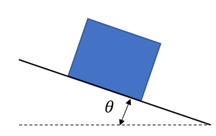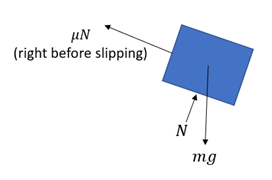## Friction on a Ramp

Consider a current equilibrium state where a box is on an inclined ramp. The static coefficient of friction between the two is 0.5. As the ramp is pivoted upwards, what angle will the box begin to slide?Hint
Free Body Diagram:Hint 2
Analyzing the forces normal to the ramp:
$$\sum F_{normal}=0=N-(mass\times gravity)cos\theta \rightarrow N=(mg)cos\theta$$$First, draw the free body diagram on the box.Analyzing the forces normal to the ramp: $$\sum F_{normal}=0=N-(mass\times gravity)cos\theta \rightarrow N=(mg)cos\theta$$$
Analyzing the forces tangent to the ramp:
$$\sum F_{tangent}=0=\mu N-(mass\times gravity)sin\theta\to N=\frac{(mg)sin\theta}{\mu}$$$Combining the two equations: $$\mu (mg)cos\theta =(mg)sin\theta$$$
$$\mu=\frac{sin\theta }{cos\theta }=tan\theta =0.5$$$$$\theta =tan^{-1}(0.5)=26.6^{\circ}$$$
26.6°